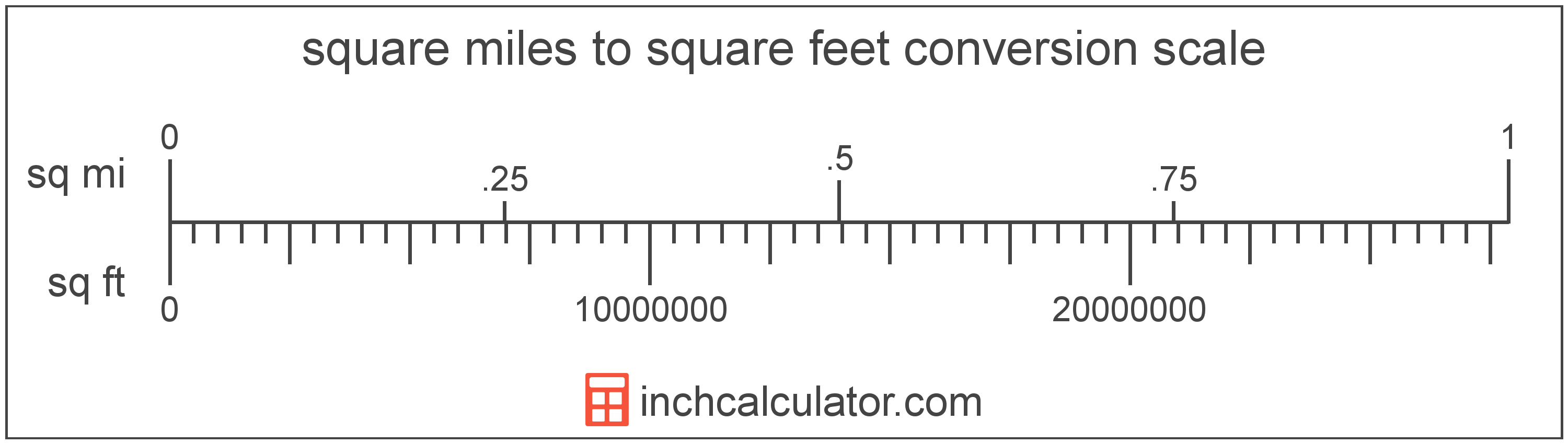# Square Miles to Square Feet Converter

Enter the area in square miles below to get the value converted to square feet.

Results in Square Feet:1 sq mi = 27,878,399.996383 sq ft

Do you want to convert square feet to square miles?

## How to Convert Square Miles to Square Feet

To convert a measurement in square miles to a measurement in square feet, multiply the area by the following conversion ratio: 27,878,399.996383 square feet/square mile.

Since one square mile is equal to 27,878,399.996383 square feet, you can use this simple formula to convert:

square feet = square miles × 27,878,399.996383

The area in square feet is equal to the area in square miles multiplied by 27,878,399.996383.

For example, here's how to convert 5 square miles to square feet using the formula above.
square feet = (5 sq mi × 27,878,399.996383) = 139,391,999.98192 sq ft### How Many Square Feet Are in a Square Mile?

There are 27,878,399.996383 square feet in a square mile, which is why we use this value in the formula above.

1 sq mi = 27,878,399.996383 sq ft

## What is a Square Mile?

One square mile is equal to the area of a square with sides that are each 1 mile long. One square mile is roughly equal to 2.59 square kilometers or 640 acres.

The square mile is a US customary and imperial unit of area. Square miles can be abbreviated as sq mi, and are also sometimes abbreviated as mi². For example, 1 square mile can be written as 1 sq mi or 1 mi².

## What is a Square Foot?

One square foot is equivalent to the area of a square with sides that are each 1 foot in length. One square foot is equal to 144 square inches or 0.092903 square meters .

The square foot is a US customary and imperial unit of area. A square foot is sometimes also referred to as a square ft. Square feet can be abbreviated as sq ft, and are also sometimes abbreviated as ft². For example, 1 square foot can be written as 1 sq ft or 1 ft².

You can use a square footage calculator to calculate the area of a space if you know its dimensions.

## Square Mile to Square Foot Conversion Table

Table showing various square mile measurements converted to square feet.
Square Miles Square Feet
0.0000001 sq mi 2.7878 sq ft
0.0000002 sq mi 5.5757 sq ft
0.0000003 sq mi 8.3635 sq ft
0.0000004 sq mi 11.15 sq ft
0.0000005 sq mi 13.94 sq ft
0.0000006 sq mi 16.73 sq ft
0.0000007 sq mi 19.51 sq ft
0.0000008 sq mi 22.3 sq ft
0.0000009 sq mi 25.09 sq ft
0.00000001 sq mi 0.278784 sq ft
0.0000001 sq mi 2.7878 sq ft
0.000001 sq mi 27.88 sq ft
0.00001 sq mi 278.78 sq ft
0.0001 sq mi 2,788 sq ft
0.001 sq mi 27,878 sq ft
0.01 sq mi 278,784 sq ft
0.1 sq mi 2,787,840 sq ft
1 sq mi 27,878,400 sq ft

## References

1. Merriam-Webster, square foot, https://www.merriam-webster.com/dictionary/square%20foot